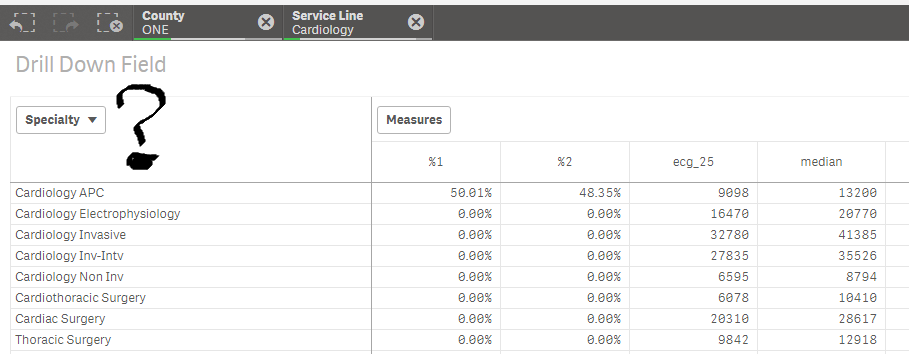Qlik Sense App Development

Discussion board where members can learn more about Qlik Sense App Development and Usage.

Announcements
QlikWorld 2020: Join us May 11 - 14, 2020 in Phoenix, AZ. Register early and save \$400. Learn More
Valued Contributor

Drill down (level) on a pivot table

Hi community,

I'm using a drill down field on a pivot table.Do you know how can I get the name of the field (level) currently being used on the pivot table?

In the above, if nothing is selected then County will be level being displayed... If only one County is selected and nothing else on the levels, then [Service Line] is supposed to be displayed... and so on. On the image above I'll need to know that "Specialty" is the field being used.

Any ideas? I thought in starting a big IF sentence to go over all levels and confirming if only one value of each is selected but I'm hoping there is a cleverer solution!

Many thanks for your help,

Cheers,

Luis

Tags (3)
1 Solution

Accepted Solutions
Valued Contributor

Re: Drill down (level) on a pivot table

So far this seems to show what the field being used should be:

IF (((GetSelectedCount(County)=1 OR GetPossibleCount(County)= 1) AND (GetSelectedCount([Service Line])=1 OR GetPossibleCount([Service Line])= 1) AND (GetSelectedCount(Specialty)=1 OR GetPossibleCount(Specialty)= 1) AND (GetPossibleCount([MD/DO vs APC])=1 OR GetSelectedCount([MD/DO vs APC])=1)),'PPEET',

IF (((GetSelectedCount(County)=1 OR GetPossibleCount(County)= 1) AND (GetSelectedCount([Service Line])=1 OR GetPossibleCount([Service Line])= 1) AND (GetSelectedCount(Specialty)=1 OR GetPossibleCount(Specialty)= 1)),'[MD/DO vs APC]',

IF (((GetSelectedCount(County)=1 OR GetPossibleCount(County)= 1) AND (GetSelectedCount([Service Line])=1 OR GetPossibleCount([Service Line])= 1)),'Specialty',

IF (((GetSelectedCount(County)=1 OR GetPossibleCount(County)= 1)),'[Service Line]','County'))))

I hope there was something more elegant...

Cheers,

Luis

Valued Contributor

Re: Drill down (level) on a pivot table

So far this seems to show what the field being used should be:

IF (((GetSelectedCount(County)=1 OR GetPossibleCount(County)= 1) AND (GetSelectedCount([Service Line])=1 OR GetPossibleCount([Service Line])= 1) AND (GetSelectedCount(Specialty)=1 OR GetPossibleCount(Specialty)= 1) AND (GetPossibleCount([MD/DO vs APC])=1 OR GetSelectedCount([MD/DO vs APC])=1)),'PPEET',

IF (((GetSelectedCount(County)=1 OR GetPossibleCount(County)= 1) AND (GetSelectedCount([Service Line])=1 OR GetPossibleCount([Service Line])= 1) AND (GetSelectedCount(Specialty)=1 OR GetPossibleCount(Specialty)= 1)),'[MD/DO vs APC]',

IF (((GetSelectedCount(County)=1 OR GetPossibleCount(County)= 1) AND (GetSelectedCount([Service Line])=1 OR GetPossibleCount([Service Line])= 1)),'Specialty',

IF (((GetSelectedCount(County)=1 OR GetPossibleCount(County)= 1)),'[Service Line]','County'))))

I hope there was something more elegant...

Cheers,

Luis